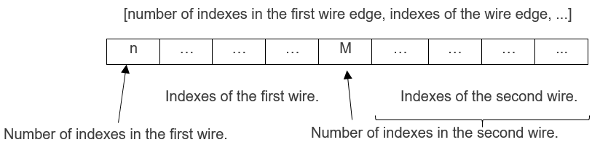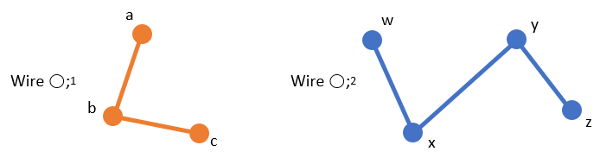# A3DTess3DWireData

## Detailed Description

struct A3DTess3DWireData

3D wire data structure

The A3DTessBaseData::m_pdCoords member represents point coordinates, which are interpreted in groups of three (X, Y, Z) to represent 3D vectors. A3DTess3DWireData enables you to organize these coordinates as wires. The A3DTess3DWireData structure uses the A3DTessBaseData::m_pdCoords array to retrieve the point coordinates. The m_puiSizesWires member contains indexes referencing the array of points in A3DTessBaseData::m_pdCoords. The index for each point is a multiple of 3 and refers to the first coordinate in the 3D point. As an example, for a single 4-point wire the m_puiSizesWires array would contain the following elements, with the first element representing the number of points in the wire and subsequent elements representing point indexes in A3DTessBaseData::m_pdCoords : {number of indexes, indexes of the wire edge} = {4, 0, 3, 6, 9}. The m_uiSizesWiresSize member represents the exact number of elements in the m_puiSizesWires array. Our single 4-point wire contains 5 elements {4, 0, 3, 6, 9}, so m_uiSizesWiresSize would be set to 5. If m_uiSizesWiresSize is set to zero, the tessellation is given as a single wire edge with the array of point indexes contained in A3DTessBaseData::m_pdCoords. If m_uiSizesWiresSize contains multiple wires, then the array referenced by m_puiSizesWires describes the edges as follows:

Version

2.0If m_uiSizesWiresSize is non-zero, m_puiSizesWires can include the flags described in Flags for Specifying Wire Characteristics as follows: [number of indexes | flag, indexes 1, indexes 2, …] m_bIsRGBA indicates the color format used is RGBA. A value of true indicates the use of RGBA, while a value of false indicates the use of RGB.m_bIsSegmentColor : A value of true indicates the colors are for the entire segment.m_pucRGBAVertices specifies the color channels for each vertex / segment.m_uiRGBAVerticesSize specifies the size of the m_pucRGBAVertices. When the colors are for points (m_bIsSegmentColor

= false), the number of colors needed must be equal to the number of points.

When the colors are for segments (

m_bIsSegmentColor

= true), the number of colors needed must be equal to the number of segments. So if a wire has 3 points, it will need 2 colors. If this 3-point wire is closed or continuous with a previous wire, it will need 3 colors.

The number of colors needed can be calculated:

```A3Duns32 iNumberColorNeeded = 0;

if (sTess3DWireData.m_bIsSegmentColor && !bIsWireClosed && !bIsWireContinuous)
{
iNumberOfColorsNeeded = sData->m_uiSize - 1;
}
else
{
iNumberOfColorsNeeded  = sData->m_uiSize;
}
```
The member m_uiRGBAVerticesSize is 3 times the number of colors needed for RGB, 4 times the number of colors needed for RGBA.
```sTess3DWireData.m_uiRGBAVerticesSize = (sTess3DWireData.m_bIsRGBA ? 4 : 3) * iNumberOfColorsNeeded;
```

Example: Consider the following example of two wires: one defined by 3 points, and another wire defined by 4 points.Note

The A3DTess3DWire entity includes data stored in the A3DTessBaseData structure.

Warning

Implicit points coming from Flags for Specifying Wire Characteristics must also bear a color.

Warning

A3DTessBaseData::m_uiCoordSize represents size of array A3DTessBaseData::m_pdCoords and not a number of 3D points.

Public Members

A3DUns32 m_uiSizesWiresSize

The size of m_puiSizesWires.

A3DUns32 *m_puiSizesWires

See explanations in Detailed Description.

A3DBool m_bIsRGBA

See explanations in Detailed Description.

A3DBool m_bIsSegmentColor

See explanations in Detailed Description.

A3DUns32 m_uiRGBAVerticesSize

The size of m_pucRGBAVertices.

A3DUns8 *m_pucRGBAVertices

See explanations above.